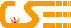Search
in english whole site
»
Dynamic clouds
A pretty cool javascript to draw fractal clouds. Try it on the fly!

A class of fractal, the "folded plan" is used to make clouds. A plan is of dimension 2. When it is folded like aluminium foils, its dimension increase between 2 and 3. Hence such objects are fractals. With adequate coloring, such folded plans can be used to create rather realistic landscapes, for instance by adding a cut-off value for the sea level. Similarly, looking at the plan from above and using the altitude as an intensity map, clouds-like shapes can be obtained.

### Fractal Clouds

 Iterations: Initial Amplitude: Perturbation: Perturbation Dampening: Wait... Calculating! javascript provided by, 2006

The algorithm
Take a square (2x2 points) and set for each point a value randomly (based on the initial amplitude).
For example:

 a c g i

Extend the square (3x3) and fill up the new values as follows:

 a b = (a+c)/2+perturbation c d = (a+g)/2+perturbation e = (a+c+g+i)/4+perturbation f = (c+i)/2+perturbation g h = (g+i)/2+perturbation i

Iteratively continue the same procedure. The next square is 5x5, then 9x9, etc... (2^iteration+1).
As an example, the 2nd iteration looks like:

 a (a+b)/2+perturbation b (b+c)/2+perturbation c (a+d)/2+perturbation (a+b+d+e)/4+perturbation (b+e)/2+perturbation (b+c+e+f)/4+perturbation (c+f)/2+perturbation d (d+e)/2+perturbation e ... f ... ... ... ... ... g ... h ... i

As the distance is lessening, the perturbation is also taken smaller. Indeed at each iteration, the perturbation is divided by the dampening.

At this point the grid can be rendered. First render a background (e.g. a light blue grandient), then draw small white squares taking the (normalized) value as transparency.

That's it!

The code (create the grid)
``` var surf=newMDA(2,2,2); // newMDA creates a bidimentional array var sz = 2; surf = multiMDA(surf,ini_ampl); // multiplies a bidimentional array by a constant var cnt = 1; for (i=0; i sz = Math.pow(2,cnt)+1; surf2 = newMDA(sz,sz,0); for (j=0;j for (k=0;k // corners copied surf2[2*j][2*k] = surf[j][k]; surf2[2*j][2*(k+1)] = surf[j][k+1]; surf2[2*(j+1)][2*k] = surf[j+1][k]; surf2[2*(j+1)][2*(k+1)] = surf[j+1][k+1]; // center surf2[2*j+1][2*k+1] = (surf[j][k]+surf[j+1][k]+surf[j][k+1]+surf[j+1][k+1])/4+bipolarRnd(0,1)*pert; // edges mids surf2[2*j+1][2*k] = (surf[j][k]+surf[j+1][k])/2+bipolarRnd(0,1)*pert; surf2[2*j+1][2*(k+1)] = (surf[j][k+1]+surf[j+1][k+1])/2+bipolarRnd(0,1)*pert; surf2[2*j][2*k+1] = (surf[j][k]+surf[j][k+1])/2+bipolarRnd(0,1)*pert; surf2[2*(j+1)][2*k+1] = (surf[j+1][k]+surf[j+1][k+1])/2+bipolarRnd(0,1)*pert; } } surf = surf2; pert /= pert_dampen; cnt = cnt + 1; } ```